Shell ScriptingLearn about Arithmetic Operators in Linux Shell Scripting

# Learn about Arithmetic Operators in Linux Shell Scripting

Variables would be completely useless if we didn’t have operations to be done with them.
But, fortunately, we do have.

The Linux Shell has rich list of operators. Operators are divided into several types:

1) Arithmetic Operators.
2) Logical Operators.
3) String Operators.
4) Comparison Operators.
5) File Test Operators.

In this article, we are going to talk about the first type (Arithmetic Operators). The types from the second to the fifth will be discussed in the next article when talking about test conditions.

Arithmetic Operators
Performing Arithmetic computations is one of the main reasons for writing computer programs. For example, you may need to calculate the factorial of an integer, the area of a circle, or the average of a group of numbers.

The following table lists the Arithmetic Operators that the Linux shell supports:

 Operator Usage/Description + Plus – adds two operands – Minus – Subtracts the second operand (on the right side of the operator) from the first. * Asterisk – Multiplies the two operands. / Division – Divides the first operand (on the left side) by the second operand. ** Exponentiation – Raises the first operand to the power of the second operand. % Modulus – Performs the division, but returns the reminder. += Plus Equal – Adds the value on the right side to the variable on left side, and stores the result in the left side variable. In other words, it increments the left side operand by the value of the right side one. -= Minus Equal – Decrements the left side operand by the value of the right side one. *= Times Equal – Multiplies the left side operand by the right side operand and stores the result in the left side variable. /= Slash Equal – Divides the left side operator by the right side one, and stores the result in the left side variable. ++ Self Increment operator. — Self Decrement operator.

Examples
Given that x=5 and y=2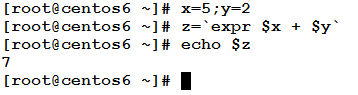This added the value of x and the value of y and stored the result in the variable z.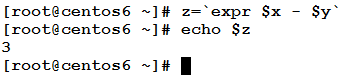This operation has subtracted y from x and stored the result in the variable z.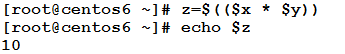The above operation has multiplied x by y and stored the result in z.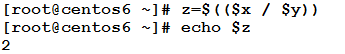This operation has divided x by y and stored the result in z.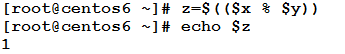This operation has divided x by y, and stored the reminder in z.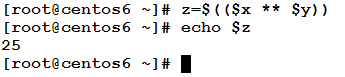This operation has raised x to the power of y, and stored the result in z.

Examples
This example illustrates the arithmetic assignment operators.
Given x=8, y=2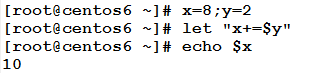This operation has incremented the variable x by the value of y.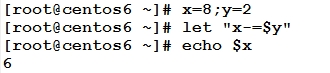This operation has decremented the variable x by the value of y.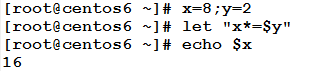This operation has multiplied x by y and stored the result in x.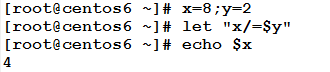This operation has divided x by y and stored the result in x.Examples
In this example, we will see how the self increment and decrement operators work.
Given counter=10.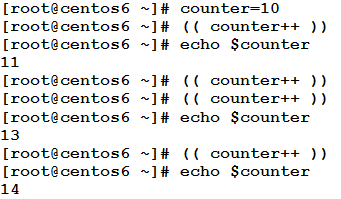The self increment operator “++” increments the value of the variable counter by 1. This is equivalent to saying:

`counter=\$((\$counter+1))`

Or:

`let "counter+=1"`

Obviously, using the self increment operator makes you type less while having the same result. Personally, I do prefer to use it.

Now, let’s try the opposite.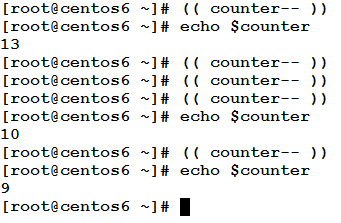* * * * * *

Example
In this example, we are going to write a script that prompts the user to enter two numbers. The script then performs the basic arithmetic operations using the two numbers, and prints their results back to the user.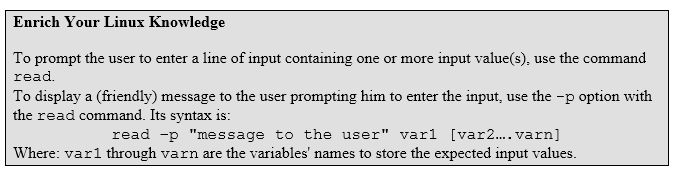The script could be something like the following:

```[[email protected] ~]# cat -n calc.sh
1  #!/bin/bash
2  # This script asks the user to enter two numbers.
3  # and performs the basic arithmetic operations.
4  # Author: Eduonix
5  # Date: Aug. 2015
6  read -p "Enter two numbers: " x y
7  A=`expr \$x + \$y`
8  S=\$((\$x - \$y))
9  M=\$((\$x * \$y))
10  D=\$((\$x / \$y))
11  let "P = \$x ** \$y"
12  echo "\$x + \$y = \$A"
13  echo "\$x - \$y = \$S"
14  echo "\$x * \$y = \$M"
15  echo "\$x / \$y = \$D"
16  echo "\$x ** \$y = \$P"
[[email protected] ~]#
```

Will that work? let’s see: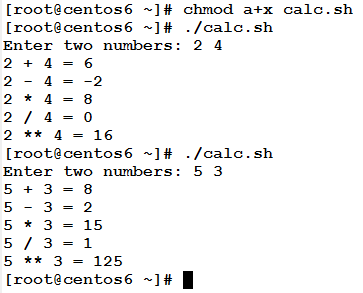Now, to the explanation:

– Line1: the Shebang statement, that tells the program loader to use the exutable /bin/bash as interpreter for this script file.
– Lines 2-5: comments that document the purpose of this script, its author, and the date it was written.
– Line 6: uses the command read –p to prompt the user to input two numbers. The inputs will be assigned to the variables x and y.
– Line 7: uses the command substitution using the back ticks “ to assign the output from the expr command to the variable A. the command expr is used to evaluate expressions. In this line, it performs an addition operation using the + operator.
– Lines 8-10: use the \$(()) to evaluate arithmetic operations.
– Line 11: uses the let command to raise x to the power of y and store the result in P.
– Lines 12-16: print the results of the arithmetic operations to the user.

* * * * * *

In this article, we have talked about the first type of operators: the Arithmetic Operators. We have investigated the various operators supported by the Linux shell, and the arithmetic operations they perform. Also, we have illustrated our talking by examples.

In the next article, we are going to talk about the remaining types of operators, and their usage in test conditions. See you there.

### Build A Front End Of an e-Commerce Site Using React

21,501Fans
4,213Followers
104,000Subscribers

### Build A Front End Of an e-Commerce Site Using React

- Advertisement -